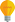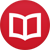# Pythagorean tuning

by Jakub MarianTip: See my list of theMost Common Mistakes in English. It will teach you how to avoid mis­takes with com­mas, pre­pos­i­tions, ir­reg­u­lar verbs, and much more.

Pythagoras devised a tuning system based solely on perfect fifths, constructed from a reference tone by successively stacking perfect fifths (i.e. the frequency ratio 3:2), shifted by one or more octaves when necessary. This tuning is traditionally understood as a way of tuning the twelve tones of the western twelve-semitone system, as in the following diagram (dashes indicate a perfect fifth):

E♭ – B♭ – F – C – G – D – A – E – B – F♯ – C♯ – G♯

This tuning produces quite satisfactory results for compositions that do not require other tones, that is, other sharps or flats, and is believed to be widely used during the medieval and renaissance periods. However, for pieces that use chromatic alterations or more complex harmonic progressions, approximating, for example, D♯ using E♭ is unsatisfactory, and so other tunings were adopted during the baroque period.

Another way to understand this tuning is that the chain of perfect fifths extends indefinitely both to the left and to the right (if you keep the 3:2 ratio, the tones never repeat, i.e. even after shifting to the same octave, D♯ and E♭ have different frequencies), whence we obtain the following line (which shall be referred to as the line of fifths):

… – D♭ – A♭ – E♭ – B♭ – F – C – G – D – A – E – B – F♯ – C♯ – G♯ – D♯ – …

The notes after B♯ would be most naturally denoted using the double sharp sign (and then “triple sharps” and so on), and similarly for the notes before F♭.

This approach is of little practical use, since a keyboard or a fretted instrument implementing such a tuning would require an infinite number of keys (resp. frets), or at least enough keys to accommodate commonly used scales with some maneuvering space for chromatic alterations, which would be over 30 keys per octave.

Impractical as it may be, the line of fifths can serve as a great tool for understanding elementary principles of tonal music and the contemporary musical notation. First thing to notice is that a diatonic scale can be constructed by successively stacking fifths. For example, C major consists of F and six fifths above it, or, alternatively, it can be obtained by taking one fifth below C (i.e. F) and five fifths above C (i.e. G D A E B).

This must be true for any major scale, so, for example, E major consists of one fifth below E (i.e. A) and five fifths above it (i.e. B F♯ C♯ G♯ D♯), as can be easily seen from the line of fifths. Using the enharmonic equivalence, the line of fifths is generally regarded as the cycle of fifths, although the latter does not reflect the notational structure (since the tones of E major would never be denoted A E B F♯ D♭ A♭ E♭).

## Using the line of fifths for harmony analysis

Another thing to notice is that the function of a note in a key is determined by its “tonal distance” from the tonal center (i.e. the number of fifths one has to move from the tonal center to reach it). Obviously, the right neighbour of the tonal center is the dominant of that key (since it is one fifth above it), the left neighbour is the subdominant of that key (since it is one fifth below it, which is the same as one fourth above it). A quick glimpse at the C-major segment of the line of fifths will provide all other harmonic functions: two steps to the right of the tonal center is the supertonic (resp. II), three steps provide the submediant (VI), four the mediant (III), and five the leading tone (VII).

In practical terms this means that to remember all scale notes together with their respective functions in a scale it suffices to remember their tonal distance from the tonal center, which in turn means to remember their relative position in the line of fifths. This alone can be much more effective than pure memorization or other traditional methods of learning (since it is possible to remember the line of fifths visually and recall it when necessary), but in fact any kind of memorization can be eliminated using an appropriate naming convention, and this is the essence of the next section.

By the way, I have written several educational ebooks. If you get a copy, you can learn new things and support this website at the same time—why don’t you check them out?